# Tkinter Pack

Summary: in this tutorial, you’ll learn about the Tkinter `pack` geometry manager and how to use it to arrange widgets on a window.

## Introduction to the Tkinter pack geometry manager

So far, you have learned how to use the `pack()` method to add widgets to a window.

To arrange widgets on a window, you use geometry managers. The `pack()` method is one of three geometry managers in Tkinter. The other geometry managers are `grid()` and `place()`.

The `pack` geometry manager has many configurations. The following are the most commonly used options: `fill``expand``side``ipadx``ipady``padx`, and `pady`.

## Tkinter pack geometry manager example

The following shows how to use the `pack` geometry manager to arrange two Label widgets on the root window:

```
```

`import tkinter as tk root = tk.Tk() root.title('Pack Demo') root.geometry("300x200") # box 1 box1 = tk.Label( root, text="Box 1", bg="green", fg="white" ) box1.pack( ipadx=10, ipady=10 ) # box 2 box2 = tk.Label( root, text="Box 2", bg="red", fg="white" ) box2.pack( ipadx=10, ipady=10 ) root.mainloop() `

Code language: Python (python)

Output: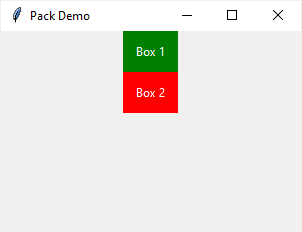This example uses the `ipadx` and `ipady` for internal padding. These options create spaces between the labels and their borders.

As you can see, the `pack` geometry manager piles the label widgets on top of each other.

### 1) Using the fill option

The fill option accepts three values `'x'``'y'`, and `'both'`. These options allow the widget to fill available space along the x-axis, y-axis, and both.

If you add the `fill='x'` to the first widget like this:

```
```

`box1.pack( ipadx=10, ipady=10, fill='x' ) `

Code language: Python (python)

… you’ll see that the widget fills all available space across the x-axis: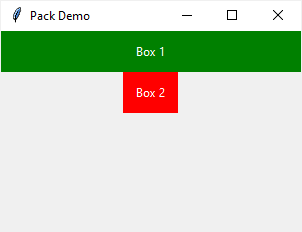However, if you change to `fill='y'` as follows:

```
```

`box1.pack( ipadx=10, ipady=10, fill='y' ) `

Code language: Python (python)

… you’ll see that the first widget doesn’t fill all space vertically: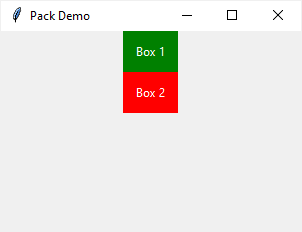Basically, the `pack` geometry manager allocates space to each widget as highlighted in the following picture: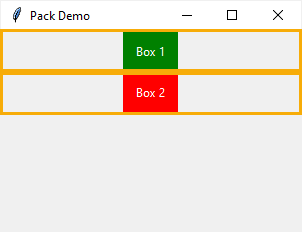When you use the `fill` option, the area of each widget can fill is constrained by those allocated areas.

### Using the expand option

The `expand` option allocates more available space to a widget.

If you add the `expand` option to the first widget:

```
```

`box1.pack( ipadx=10, ipady=10, expand=True ) `

Code language: Python (python)

…you’ll get the following output: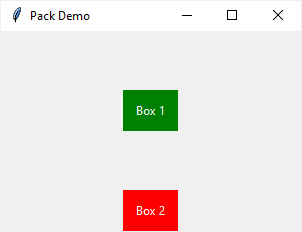The first widget takes all available space in the window except for the space allocated to the second widget.

Since the first widget doesn’t have the `fill` option, it floats in the middle of the allocated area.

If you set `fill` to `'both'`:

```
```

`box1.pack( ipadx=10, ipady=10, fill='both', expand=True ) `

Code language: Python (python)

…you’ll see that the first widget fills up most of the window like this: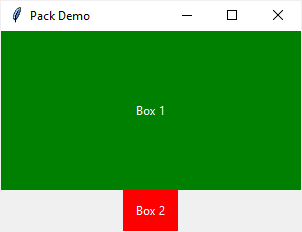If you add the `expand` option to both widgets, the `pack` manager will allocate the space to them evenly. For example:

```
```

`import tkinter as tk root = tk.Tk() root.title('Pack Demo') root.geometry("300x200") # box 1 box1 = tk.Label( root, text="Box 1", bg="green", fg="white" ) box1.pack( ipadx=10, ipady=10, expand=True, fill='both' ) # box 2 box2 = tk.Label( root, text="Box 2", bg="red", fg="white" ) box2.pack( ipadx=10, ipady=10, expand=True ) root.mainloop() `

Code language: Python (python)

Output: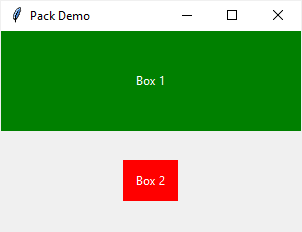Notice that the second widget doesn’t use all allocated space because it doesn’t have the `fill` option.

When you set the `expand` to `True` for all widgets, the `pack` manager will allocate space to them evenly.

However, this is only true when all the widgets share the same anchor `side`.

### Using side option

The `side` option specifies the alignment of the widget. It can be `'left'``'top'``'right'`, and `'bottom'`.

The `side` defaults to `'top'`. In other words, widgets are aligned to the top of their container.

The following example sets the `side` of the first widget to `'left'`:

```
```

`import tkinter as tk root = tk.Tk() root.title('Pack Demo') root.geometry("300x200") # box 1 box1 = tk.Label( root, text="Box 1", bg="green", fg="white" ) box1.pack( ipadx=10, ipady=10, expand=True, fill='both', side='left' ) # box 2 box2 = tk.Label( root, text="Box 2", bg="red", fg="white" ) box2.pack( ipadx=10, ipady=10, expand=True, fill='both' ) root.mainloop() `

Code language: Python (python)

Output: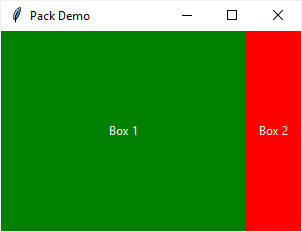In this example, the `expand` option may not work as you expected. The reason is that widgets have different sides.

To make their space even again, you can set the `side` of both widgets to `'left'` or one is `'left'` and the other is `'right'`:

```
```

`import tkinter as tk root = tk.Tk() root.title('Pack Demo') root.geometry("300x200") # box 1 box1 = tk.Label( root, text="Box 1", bg="green", fg="white" ) box1.pack( ipadx=10, ipady=10, expand=True, fill='both', side='left' ) # box 2 box2 = tk.Label( root, text="Box 2", bg="red", fg="white" ) box2.pack( ipadx=10, ipady=10, expand=True, fill='both', side='left' ) root.mainloop() `

Code language: Python (python)

Output: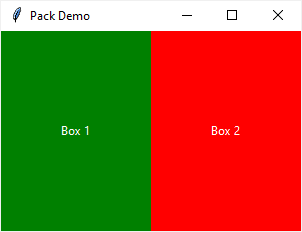## When to use the pack geometry manager

The geometry manager is suitable for the following:

• Placing widgets in a top-down layout.
• Placing widgets side by side

See the following example:

```
```

`import tkinter as tk from tkinter import ttk root = tk.Tk() root.title('Pack Demo') root.geometry("300x200") # place widgets top down label1 = tk.Label( root, text='Box 1', bg="red", fg="white" ) label1.pack( ipadx=10, ipady=10, fill='x' ) label2 = tk.Label( root, text='Box 2', bg="green", fg="white" ) label2.pack( ipadx=10, ipady=10, fill='x' ) label3 = tk.Label( root, text='Box 3', bg="blue", fg="white" ) label3.pack( ipadx=10, ipady=10, fill='x' ) # place widgets side by side label4 = tk.Label( root, text='Left', bg="cyan", fg="black" ) label4.pack( ipadx=10, ipady=10, expand=True, fill='both', side='left' ) label5 = tk.Label( root, text='Center', bg="magenta", fg="black" ) label5.pack( ipadx=10, ipady=10, expand=True, fill='both', side='left' ) label6 = tk.Label( root, text='Right', bg="yellow", fg="black" ) label6.pack( ipadx=10, ipady=10, expand=True, fill='both', side='left' ) root.mainloop() `

Code language: Python (python)

Output: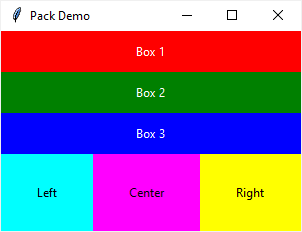## Summary

• Use Tkinter pack geometry manager to arrange widgets in a top-down layout or side by side.
• Use the `fill``expand`, and `side` options of pack geometry manager to control how widgets arranged.